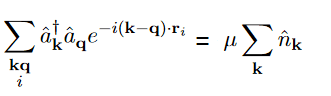# Kronecker delta by using creation/annihilation operators

• I
Faust90
Hey all,

i've found the following expression:How do they get that? They somehow used the kronecker delta Sum_k exp(i k (m-n))=delta_mn. But in the expression above, they're summing over i and not over r_i??

Best

Faust90
Do you need more background or is the question not precise enough? :-)

Mr-R
Hi Faust90

The index ##i## under the sum refers to the subscript ##i## under the ##r##. The other i is the imaginary number. The Kronecker delta gets rid of the exponential and thus the sum on ##i## anyway. Try this website and see if it clears any confusion you are having. http://www.physicspages.com/2014/11/09/discrete-fourier-transforms/

Faust90
Hi Mr-R,

thanks for your answer. Yes, but my problem is that the sum is not running over r_i but over i.
Let's assume the r_i are an set of positions, for example always the same position, i.e. r_i={1,1,1,1,1,1,....}. Then in the end, that's just a product
prod_n=0^\infity e^{i(k-q)}

Best,